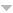# Publications

Found 169 results
[ Author] Title Type Year
Filters: First Letter Of Last Name is Y  [Clear All Filters]
Y
Index integral transformations of Titchmarsh type. J. Comput. Appl. Math.. 1997;85:169-179.
On the Yor integral and a system of polynomials related to the Kontorovich-Lebedev transform. Integral Transforms Spec. Funct.. 2013;24:672-683.
A general class of Voronoi's and Koshliakov-Ramanujan's summation formulas involving \$d_k(n)\$. Integral Transforms Spec. Funct.. 2011;22:801-821.
A remark on the inversion formula for Wimp's integral transformation with respect to the index. Differentsial\cprime nye Uravneniya. 1985;21:1097-1098, 1104.
On the Weber integral equation and solution to the Weber–Titchmarsh problem. Journal of Mathematical Analysis and Applications. 2018;460(1):400-410.
A new Kontorovich-Lebedev-like transformation. Commun. Math. Anal.. 2012;13:86-99.
Composition theorems of Plancherel type for index transformations. Dokl. Akad. Nauk Belarusi. 1994;38:29-32, 122 (1995).
A class of integral equations and index transformations related to the modified and incomplete Bessel functions. J. Integral Equations Appl.. 2010;22:141-164.
A real inversion formula for the bilateral Laplace transform. Izv. Nats. Akad. Nauk Armenii Mat.. 2005;40:67-79.
Integral equations and convolutions associated with transformations of Kontorovich-Lebedev type. Differentsial\cprime nye Uravneniya. 1993;29:1272-1284, 1288.Edit
Uncertainty principles for the Kontorovich-Lebedev transform. Math. Model. Anal.. 2008;13:289-302.
Boundedness and inversion properties of certain convolution transforms. J. Korean Math. Soc.. 2003;40:999-1014.
The Titchmarsh integral transformation by the index of a Bessel function. J. Comput. Appl. Math.. 2000;118:353-361.
Generalizations of the Leibniz rule to integral convolutions. Dokl. Akad. Nauk BSSR. 1991;35:111-115, 188.Edit
Lebedev's type index transforms with the modified Bessel functions. Commun. Math. Anal.. 2016;19(2):68-81.
About a new class of integral transforms in Hilbert space. Math. Balkanica (N.S.). 1995;9:179-191.
The use of the Kontorovich-Lebedev transform in an analysis of regularized Schrödinger equation. Integral Transforms Spec. Funct.. 2013;24:9-22.Edit
A radial version of the Kontorovich-Lebedev transform in the unit ball. Opuscula Math.. 2011;31:137-146.Edit